## Part 7: Map Processing

I've talked about it before, but I really didn't take an in-depth look at it. So, for everyone's enjoyment (I hope), the map processing algorithm in full detail! And with comments.

## Maps

Maps are stored in a data array located in the lower 8k, at address >2000->3FFF. I decided to allocate 4 kilobytes of space for maps, which would allow a maximum size of 64x64 square, or 4096 individual tiles.

The data is stored as a linear array, but in practice, it can be read in different ways. For example, there could be only 32 columns, but 128 rows. In the buffer, it makes no difference, it's only in map processing that the map's true form is determined.

One particular form that won't be in the demo but in the final version is slanted maps. I came up with the idea when trying to design a coastline area. it was frustrating to have to make the coast conform to a square or rectangle, which was fairly obvious in the layout.

Then I thought about it and realized, I could set up the map processing to slant the rows either to the left or right, creating a diagonal map. It will be more work to compensate for the changes necessary, but the end result should be worth it. For now, that part of the code is partially implemented but not tested.

## Building the Map View

There are five stages to map processing:

• Extract the map data from the buffer to a temporary workspace map
• Calculate the mobile objects (Mobs) and place them on the temp map
• Calculate lighting on the map
• Calculate line-of-sight on the map
• Output the map to the screen
All of this adds up into a considerable amount of work, and I'm proud to say that the TI can do all this work in a fraction of a second and have it on screen in real-time.

### Step One

The first part is extracting the map from the buffer, which has some pitfalls. Here's a code excerpt:

```VWIDTH BSS  2			Vertical width of map
HWIDTH BSS  2			Horizontal width of map
Y      BSS  2			Y coordinate of player
X      BSS  2			X coordinate of player
DIRX   DATA 0,-1,0,1		Directional vectors
DIRY   DATA 1,0,-1,0

* Extract map from map buffer
MOV  @Y,R1		Move Y coordinate into R1
MOV  @X,R2		Move X coordinate into R2
AI   R2,-6
LI   R3,13		Set R3 and R4 to 13 (window boundaries
LI   R4,13
CLR  R5			Clear R5 and R6
CLR  R6
MOVB @STATE+5,R6		Copy map type (slant or normal) into R6 (0, 1, or 2)
SRL  R6,7		Set R6 to 0, 2, or 4
MOV  @DIRY(R6),R8	Move X-offset for map type into R8 (-1, 0, or 1)
MOV  R8,R12		Copy X-offset into R12
BM1    C    R1,@VWIDTH		Check if Y is out of bounds
JHE  BM2			Check unsigned value (negative is higher as well)
MOV  R1,R6		Copy R1 (Y value) into R6
BM15   C    R2,@HWIDTH		Check if Y is out of bounds
JHE  BM3			Check unsigned value
MPY  @HWIDTH,R6		Multiply HWIDTH by R6 (Y value) R7 contains index point
A    R2,R7		Add R2 (X value) to R7 (index)
JMP  BM4
BM2    C    R1,@ZERO		Check if R1 is less than zero
JLT  BM25
MOVB @STATE+4,@STATE+4	Check @STATE+4 (map edge style) for zero (extend edge)
JEQ  BM21
MOV  R1,R6		Copy R1 (Y value) into R6
S    @VWIDTH,R6		Subtract VWIDTH from R6 (loop around)
JMP  BM15
BM21   MOV  @VWIDTH,R6		Copy VWIDTH into R6
DEC  R6			Decrement it by one (last row)
JMP  BM15
BM25   MOVB @STATE+4,@STATE+4	Check @STATE+4 (map edge style)
JEQ  BM26
MOV  @VWIDTH,R6		Copy VWIDTH into R6
A    R1,R6		Add R1 (Y value) to R6 (loop around)
JMP  BM15
BM26   CLR  R6			Set R6 to 0 (first row)
JMP  BM15
BM3    MPY  @HWIDTH,R6		Multiply HWIDTH by R6 (Y value) R7 contains index point
MOVB @STATE+4,@STATE+4	Check @STATE+4 (map edge style)
JNE  BM35
C    R2,@ZERO		Check if R2 (x value) is zero
JLT  BM4
A    @HWIDTH,R7		Add HWIDTH to R7 (index)
DEC  R7			Decrement R7 by one (last column)
JMP  BM4
BM35   C    R2,@ZER0		Check if R2 (X value) is zero
JLT  BM36
S    @HWIDTH,R7		Subtract HWIDTH from R7 (index)
JMP  BM39
BM36   A    @HWIDTH,R7		Add HWIDTH to R7 (index)
BM39   A    R2,R7		Add R2 (X value) to R7 (index)
BM4    MOVB @MAPBUF(R7),R9	Copy value in map buffer at R7 (index) into R9
MOVB R9,@WORK(R5)	Copy R9 (tile code) into work buffer at index R5 (buffer index)
SOCB @B128,@WORK(R5)	Set the eighth bit in the work buffer value just moved
ANDI R9,>8000		Convert tile in R9 to either 0 (not lit) or 128 (lit)
MOVB R9,@WORK+169(R5)	Copy light value of tile to second work buffer at WORK+169
INC  R5			Increment R5 (temp map index)
INC  R2			Increment R2 (X value)
DEC  R3			Decrement R3 (column tracking)
JNE  BM1
INC  R1			Increment R1 (Y value)
MOV  @X,R2		Copy X value into R2
AI   R2,-6		Add window offset to R2 (X value)
A    R12,R2		Add total X-offset for slant to R2 (X value)
A    R8,R12		Increment X-offset
LI   R3,13		Set R3 to 13 (column tracking)
DEC  R4			Decrement R4 (row tracking)
JNE  BM1
```
Gosh, not as easy as it sounded!

The major calculation here is determining what index in the map buffer goes to the index buffer in the temporary map buffer. Normally this would be trivial. The real issue is what to do when the position calculated is out of bounds. I have two map variants for edge processing.

One is to simply round to the nearest edge. This means the first and last rows and columns are projected in the off-buffer area, creating an illusion of an endless stretch. This technique is used on maps where I want to be able to leave the map by moving off-boundary.

The second is to repeat the map from the other side, the "wrap" effect. This lets me create some interesting maps with maze-like qualities, and also lets me break the "square" boundaries by having maps that cross over and around, creating the illusion of a non-square path. It also means the map is "closed", and you would not be able to leave unless I put an exit object somewhere.

There's also the slanting maps to consider. I haven't tested this code yet with those maps, but I've started the rudimentary work to do so. In particular, it stores the offset for each row and applies it to recalculate the position of each row in the buffer. (Maps only slant left or right, not up or down.)

Notice also that I don't have one but two temporary workspaces. The first, from WORK to WORK+168, stores the raw map data, while WORK+169 to WORK+335 is the mask upon which the raw data will eventually be applied. The SOCB instruction sets the eighth bit in each tile of the first workspace, so it's "lit" initially. In the second workspace, I use the AND to only store the eighth bit alone of each tile. This is an indicator of whether or not this tile was lit on the original map.

Although the routine works pretty fast, there are a couple potential optimizations here.

One would be to seperate the two edge checkings into two seperate routines, so that the map type (In @STATE+4) is only checked once rather than on every tile. However, this would double the memory footprint of this section, and the resulting reduction in time may be in the order of 3000 microseconds, at a loose estimate. That's about one-fifth of a sixtieth of a second. Worth the trade-off? Probably not at this stage.

### Step Two

The next step is to place the mobile objects, or mobs, which are stored in eight-byte structured array in MOBBUF. My game allows a maximum of 64 for any single map, and they're stored individually in records for flexibility. Note that R8 (the slant offset) is carried over from the prior stage.

```* Place mobs onto screen map
SETO @STATE+10			Set all values in STATE+10 to STATE+17 to >FF
SETO @STATE+12			These store the adjacent tiles and mobs
SETO @STATE+14
SETO @STATE+16
CLR  R1				Clear R1 (mob index)
MOV  @STATE+6,R0			Check if STATE+6 (mob count) is zero
JEQ  BM7				If so, skip this stage entirely
BM5    MOVB @MOBBUF(R1),@MOBBUF(R1)	Check mob type for zero
BM51   A    @W8,R1			Increment mob index by 8
DEC  R0				Decrement R0 (mob count)
JNE  BM5				If not zero, repeat
JMP  BM7				End stage
* Determine if mob is in visible window
BM6    MOVB @MOBBUF+3(R1),R2		Copy Y value (offset 3 in MOBBUF at index R1) into R2
SRL  R2,8			Convert R2 to a word value
MOV  R2,R3			Copy R2 to R3
S    @Y,R2			Subtract Y (player Y coordinate) from R2
ABS  R2				Make R2 an absolute value
C    R2,@W6			Check if R2 > 6 (outside window boundaries)
JGT  BM51			If so, move on to next mob
MPY  R8,R3			Multiply R8 (offset X) by R3 (Y value)
MOV  @MOBBUF+2(R1),R2		Copy X value (offset 2 in MOBBUF at index R1) into R2
SRL  R2,8			Convert R2 to a word value
A    R4,R2			Add X-offset to R2
S    @X,R2			Subtract X (player X coordinate) from R2
ABS  R2				Make R2 an absolute value
C    R2,@W6			Check if R2 > 6
JGT  BM51			If so, move on to next mob
* Place mob at appropriate index
MOV  @MOBBUF+2(R1),R2		Move X & Y into R2
MOV  R2,R3			Copy R2 into R3
SRL  R2,8			Shift R2 to the right (X coordinate in word form)
A    R4,R2			Add X-offset to R2
ANDI R3,>00FF			Mask R3 (Y coordinate in word form)
S    @Y,R3			Subtract Y (player Y coordinate) from R3
S    @X,R2			Subtract X (player Y coordinate) from R2
MPY  @W13,R3			Multiply R3 by 13 (Window width)
A    R2,R4			Add R2 to R4 (window index of mob)
AI   R4,84			Add 84 to R4 (window index of mob)
* Check is mob is adjacent to player
MOV  R1,R5			Copy R1 (mob index) into R5
SLA  R5,5			Shift index value to high-byte, divided by 8
CI   R4,97			Check if R4 is 97 (adjacent south)
JNE  BM61
MOVB R5,@STATE+11		Copy R5 (mob number) into STATE+11
JMP  BM64
BM61   CI   R4,83			Check if R4 is 97 (adjacent left)
JNE  BM62
MOVB R5,@STATE+13		Copy R5 (mob number) into STATE+13
JMP  BM64
BM62   CI   R4,71			Check if R4 is 71 (adjacent north)
JNE  BM63
MOVB R5,@STATE+15		Copy R5 (mob number) into STATE+15
JMP  BM64
BM63   CI   R4,85			Check if R4 is 97 (adjacent right)
JNE  BM64
MOVB R5,@STATE+17		Copy R5 (mob number) into STATE+17
BM64   MOVB @MOBBUF+1(R1),@WORK(R4)	Copy mob graphic into map buffer
JMP  BM51
BM7    MOVB @WORK+97,@STATE+10		Set STATE+10 to tile south of player
MOVB @WORK+83,@STATE+12		Set STATE+12 to tile west of player
MOVB @WORK+71,@STATE+14		Set STATE+14 to tile north of player
MOVB @WORK+85,@STATE+16		Set STATE+16 to tile east of player
MOVB @WORK+84,@STATE+18		Set STATE+18 to tile under player
```
In order to guarantee speed when the player tries to move about, I handle some pre-processing at this stage. The memory area from STATE+10 to STATE+17 stores the contents of the squares immediately adjacent to the player at the center. The even addresses store the actual tiles located there, while the odd addresses contain the mob number of any mob that is located adjacent, if any. Because zero is a legitimate mob value, I use >FF to indicate that there is no mob present.

By doing this, I can instantly determine if the player can even move in a given direction (by checking the tile value's block bit) or if moving in a particular direction may generate a mob event.

A particular concern with placing mobs is determining their relative position on the temp map, based on their coordinates. In the end, I decided to calculate the positions based upon their proximity to the player, at the center. This works out to be much easier than re-calculating map indices.

First, I check that they're in visible range (within six tiles) in both the X and Y axis. (Compensating on the X-axis if the map is slanted.) Then, if they are, I can do the work of calculating their exact index position on the map. And finally, they're placed. If their position is adjacent to the player, the values in the STATE array are updated.

### Step Three

Now we move on to lighting, something I'd planned on, but wasn't sure I could pull off:

```LIGHT  DATA >7777,>7666,>7777,>7777	Data mask for lighting
DATA >6655,>5667,>7777,>6554
DATA >4455,>6777,>6554,>3334
DATA >5567,>7654,>3222,>3456
DATA >7654,>3221,>2234,>5665
DATA >4321,>0123,>4566,>5432
DATA >2122,>3456,>7654,>3222
DATA >3456,>7765,>5433,>3455
DATA >6777,>6554,>4455,>6777
DATA >7766,>5556,>6777,>7777
DATA >7666,>7777,>7000

* Lighting Algorithm
MOVB @STATE+8,@STATE+8		Check if STATE+8 (map light level) is zero (fully lit)
JEQ  LOS				If so, skip this stage
* Check party light level, map onto tiles
MOVB @STATE+9,@STATE+9		Check if STATE+9 (player light) is zero
JEQ  LOS				If so, skip this stage
CLR  R1				Clear R1 (map index)
LI   R5,169			Set R5 to 169 (map counter)
LGT1   MOV  R1,R3			Copy R1 to R3 (light map index)
SRL  R3,1			Divide R3 by 2 (light map index)
MOVB @LIGHT(R3),R2		Copy value in LIGHT at index R3 into R2
COC  @W1,R1			Check if R1 is even (0) or odd (1)
JEQ  LGT11
SRL  R2,4			Shift value in R2 4 bits (even offset)
LGT11  ANDI R2,>0F00			Mask out all but the relevant part of the light map
SB   @STATE+9,R2			Subtract STATE+9 (player light level) from R2
JGT  LGT12			If not zero or less, move on
MOVB @B128,@WORK+169(R1)		Set value in second map buffer to >80 (lit)
LGT12  INC  R1				Increment R1 (map index)
DEC  R5				Decrement R5 (map counter)
JNE  LGT1
```
This one's pretty short, because most of the hard work was done prior in the map buffering phase. The one new bit of calculation here is the amount of area exposed by the player's light source, if any.

All the maps have a "light map", which is stored in the eighth bit of every tile. Since I only have 128 different potential tiles on a map, I had a spare bit left over, and it's a good use for it. If the bit is 0, the square is dark, otherwise it's lit. I'll eventually create a "light editor" program to allow me to change the lighting levels on maps dynamically, but for now, I just used a hex editor to darken the maps in the appropriate places.

There's two initial checks which skip the whole process. The @STATE+8 byte stores whether or not the map is "dark" at all, and this section is skipped if that is the case. The @STATE+9 byte stores if the players have an active light source, and again the section is skipped if they don't.

If a player has a light source, then the radius, stored in @STATE+9, is considered. A light map is stored in the @LIGHT array, consisting of 169 4-bit numbers, from 0-15. This is a mask onto the map view, forming a series of radial circles around the center position, the outer rings being higher value than the inner rings.

The light radius value is subtracted from the light map's value. If the result is equal or lower than zero, the square is lit. Otherwise, it remains in whatever state it's in.

I was a little worried that the extra processing may slow down the map, but it actually speeds things up quite a bit! Because a lack of light blocks out large portions of the map, LOS calculations are reduced. I will need to build in an delay system so that you always travel at a minimum speed, regardless of how "quick" map processing goes.

I also had another fiendishly clever idea... since 0 indicates a lit map, I can use the value found at @STATE+8 to also indicate a rate of speed at which lights burn out, or even a reduction in radius, to simulate very dark dungeons... Bring plenty of torches.

### Step Four

And now, the 800-pound gorilla of the routine, the LOS algorithm:

```XDIFF  BYTE 1,1,1,1,1,1,0,-1		LOS Array for movement towards center
BYTE -1,-1,-1,-1,-1
BYTE 6,5,4,3,2,1,0,1
BYTE 2,3,4,5,6
DIRX   DATA 0,-1,0,1		Directional vectors
DIRY   DATA 1,0,-1,0

* Los Algorithm
LOS    LI   R5,168			Set R5 to 168 (end of map buffer)
LI   R1,12			Set R1 to 12 (window Y counter)
LI   R2,12			Set R2 to 12 (window X counter)
CLR  R8				Clear R8 (path flag)
LOS1   LI   R9,WORK+338			Set R9 to point to WORK+338 (buffer for path indices)
CLR  R12				Clear R12 (path counter)
MOV  R5,*R9+			Move current index into R9 buffer
INC  R12				Increment path counter
MOV  R1,R3			Copy R1 to R3
MOV  R2,R4			Copy R2 to R4
MOVB @WORK+169(R5),R0		Copy present value at index in second map buffer to R0
CB   @SPACE,R0			Check if it's dark (a space)
CB   @B1,R0			Check if it's been traversed (>01)
LOS11  MOV  R8,R8			Check path flag
BL   @POSCL2			Otherwise, do second path
JMP  LOS13
LOS12  BL   @POSCLC			Do first path
LOS13  INC  R12				Increment path counter
CI   R7,84			Check if center has been reached
JNE  LOS15			If not, goto LOS15
LOS14  DECT R9				Decrement R9 by 2
MOV  *R9,R4			Move value at R9 buffer into R4
MOVB @B1,@WORK+169(R4)		Copy >01 into second map buffer at index R4
DEC  R12				Decrement R12 (path counter)
JMP  LOS18
LOS15  MOVB @WORK(R7),R6		Get the actual tile from first map buffer into R6
SRL  R6,8			Make R6 an unsigned word value
ANDI R6,>007F			Mask out the high bit at >0080
MOVB @TILES(R6),R0		Copy the tile stats at index R6 into R0
ANDI R0,>8000			Check if the high-bit is set
JNE  LOS16			If not, goto LOS16
JMP  LOS11			Otherwise, goto LOS11
LOS16  MOV  R8,R8			Check path flag
INC  R8				Increment R8
JMP  LOS1
LOS17  MOVB @SPACE,@WORK+169(R5)	Copy >20 into second map buffer at current index
LOS18  DEC  R5				Decrement R5 (buffer index)
CLR  R8				Clear R8 (path flag)
DEC  R2				Decrement R2 (X position)
CI   R2,-1			Check if R2 is -1
JGT  LOS1			If so, goto LOS1
LI   R2,12			Set R2 to 12
DEC  R1				Decrement R1 (Y position)
CI   R1,-1			Check if R1 is -1
JGT  LOS1			If so, goto LOS1

* LOS Subroutines
POSCLC MOVB @XDIFF(R3),R6		Copy direction vector of R3 (Y coord) into R6
MOVB @XDIFF(R4),R7		Copy direction vector of R4 (X coord) into R7
SRA  R6,8			Set R6 to a signed word value
SRA  R7,8			Set R7 to a signed word value
A    R6,R3			Add R6 to R3
A    R7,R4			Add R7 to R4
MOV  R3,R6			Copy R3 to R6
MPY  @W13,R6			Multiply R6 by 13
A    R4,R7			Add R4 to R7 (now the buffer map index)
MOV  R7,*R9+			Move R7 (buffer map index) to stack at R9
RT

POSCL2 MOVB @XDIFF+13(R3),R6		Copy position vector of R3 (Y coord) into R6
MOVB @XDIFF+13(R4),R7		Copy position vector of R4 (X coord) into R7
CB   R7,R6			Check if R6 is equal to R7
JL   PSC2			If R7 (X) is lower than R6 (Y), jump to PSC2
MOVB @XDIFF(R4),R6		Copy direction vector of R4 (X coord) into R6
SRA  R6,8			Set R6 to a signed word value
A    R6,R4			Add R6 to R4 (X coordinate)
JMP  PSC3
PSC1   MOVB @XDIFF(R3),R6		Copy direction vector of R3 (Y coord) into R6
MOVB @XDIFF(R4),R7		Copy direction vector of R4 (X coord) into R7
SRA  R6,8			Set R6 to a signed word value
SRA  R7,8			Set R7 to a signed word value
A    R6,R3			Add R6 to R3
A    R7,R4			Add R7 to R4
JMP  PSC3
PSC2   MOVB @XDIFF(R3),R6		Copy direction vector of R3 (Y coord) into R6
SRA  R6,8			Set R6 to a signed word value
A    R6,R3			Add R6 to R3 (Y coordinate)
PSC3   MOV  R3,R6			Copy R3 to R6
MPY  @W13,R6			Multiply R6 by 13
A    R4,R7			Add R4 to R7 (now the buffer map index)
MOV  R7,*R9+			Copy R7 (buffer map index) to stack at R9
RT
```
The algorithm of LOS processing is simple in form, but a major crunch of cycles in execution. It calculates two different paths (done in the POSCLC and POSCL2 subroutines) from each square towards the center.

The second map buffer, at this point, is a binary map; each square either contains a zero (dark, don't bother), or 128 (lit square). It follows the paths calculated in POSCLC and POSCL2, which uses a data array to determine the X and Y vectors.

If at any point it runs into a blocking tile (data for the tiles is stored in TILES, in the eighth bit), it restarts with the second path. If that's blocked as well, it blacks out (with a space character) the original square. If it reaches the center square, the square is left "open", and it "trailblazes" the successful path it followed by setting the tile values to 1.

If you consider the number of compares factored, this routine really weighs in heavy. There's 168 tiles on the screen. (not counting the center) The closer they are to the center, the less calculations are needed to reach the center. So you can figure it out like so:
• Distance of 6 = 13 + 13 + 11 + 11 = 48
• Distance of 5 = 11 + 11 + 9 + 9 = 40
• Distance of 4 = 9 + 9 + 7 + 7 = 32
• Distance of 3 = 7 + 7 + 5 + 5 = 24
• Distance of 2 = 5 + 5 + 3 + 3 = 16
• Distance of 1 = 3 + 3 + 1 + 1 = 8

• 48 * 6 (distance) * 2 (two paths) = 576
• 40 * 5 (distance) * 2 (two paths) = 400
• 32 * 4 (distance) * 2 (two paths) = 256
• 24 * 3 (distance) * 2 (two paths) = 144
• 16 * 2 (distance) * 2 (two paths) = 64
• 8 * 1 (distance) * 1 (only one path) = 8

• Total = 1448 computations (worst-case scenario)
We can discount the extra path with a distance of one, because the first tile encountered is always the center. So as far as LOS is concerned, the 8 tiles around the center are ALWAYS open. (Lighting can black them out, though.)

The worst case scenario is if all the square are unblocked except the eight surrounding the center. Unfortunately, the nature of my maps make this a likely occurence. (Like entering a small grove of forest in the middle of a plain.)

I've experimented with a sort of "reverse" trailblazing... if it finds an obstacle on a path, then technically that's a "dead-end" path and could be skipped by future traversals. But I haven't really sat down and figured out if that would work. My initial experiments have not been successful.

Another idea I've had is to change the path through the map buffer into a true "spiral" towards the center. This would, presumably, be more efficient because the farthest edges would be dealt with first all around, and any trail-blazing would benefit all the following traversals. However, this is a lot of trouble to set up, and I don't think the return is worth the investment.

A potential optmization is to re-integrate the POSCLC and POSCL2 routines, removing them as "subroutines". The main reason to do this is the overhead of using BL (Branch and Link) for every computation. Taking them out, though, would maybe net me a couple dozen cycles per computation... and make the code more difficult to maintain.

A lot of professional compilers, when faced with a large number of looping calculations, perform an optimization called "unrolling the loops". What this means is that the calculations are done in a sequential manner instead of a looping form.

Let's look at some example code. I provide the cycle counts, worst-case scenario (On the TI, that's the slow 8-bit bus memory for both registers and memory addresses.):
```LOOP   MOVB *R1+,*R2+		44 cycles
DEC  R3			22 cycles
JNE  LOOP		14 cycles
```
Not so bad, right? Well, let's say that R3 was initialized to 1024. So that's (44 + 22 + 14) * 1024 cycles to run, or 81,920 cycles.

Now let's look at the same loop, unrolled a little:
```LOOP   MOVB *R1+,*R2+		44 cycles
MOVB *R1+,*R2+		44 cycles
MOVB *R1+,*R2+		44 cycles
MOVB *R1+,*R2+		44 cycles
MOVB *R1+,*R2+		44 cycles
MOVB *R1+,*R2+		44 cycles
MOVB *R1+,*R2+		44 cycles
MOVB *R1+,*R2+		44 cycles
AI   R3,-8		30 cycles
JNE  LOOP		14 cycles
```
So in this case, we only loop 128 times, so our calculations are (396 * 128), or 50,688 cycles. Wow! That's nearly a 40% improvement in cycle use.

For my LOS algoritm, though, the looping is not so trivial. Changing POSCLC and POSCL2 so that they iteratively go through the entire path (six steps) in code to the center would probably not result in a magically huge improvement in cycle use. Besides, it's not the path routine that's eating up the cycles, it's the number of paths being calculated. This is the REAL reduction of looping we're looking for.

For example, there's the fact that diagonal and cardinal paths are essentially calculated twice, when they really only have one path. There's eight tiles in each ring like this, so let's see how many computations that costs us in redundancy:
• 8 * 6 + 8 * 5 + 8 * 4 + 8 * 3 + 8 * 2 = 48 + 40 + 32 + 24 + 16 = 160 calculations
Well, it could be worse. But trying to encode a "skip" check may end up adding too much extra work to ALL the calculations to make it worthwhile.

There's always, of course, the potential of finding a different LOS algorithm which is less processing-intensive. So far, no one's come forward with one. I may try to adapt the algorithm used in the PC versions of Ultima 4 at some point. It's slightly different than the ones used on the 8-bit platforms.

### Step Five

The final act of the map processing routine is to do the masking on the work maps and produce the final map results. Also note it places the player graphic in the center at the end:

```* Final opening of permitted space
PCMEND CLR  R5				Clear R5 (map index)
LI   R1,169			Set R1 to 169 (map index counter)
PCME1  CB   @SPACE,@WORK+169(R5)	Check for a space
MOVB @WORK(R5),@WORK+169(R5)	Copy tile from first buffer to second buffer
PCME2  INC  R5				Increment R5 (map index)
DEC  R1				Decrement R1 (map counter)
JNE  PCME1			If not zero, loop to PCME1 and repeat
MOVB @B247,@WORK+253		Copy player graphic to WORK+253 (center of map)
```
And there you have it, the map processing routine!

## Screenshots

Here's a look at some screen shots of the map processing in action: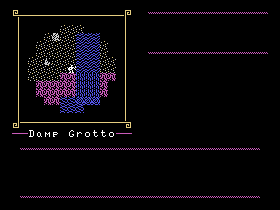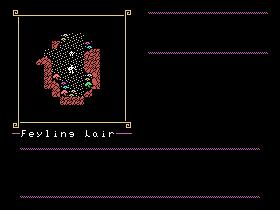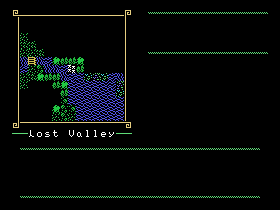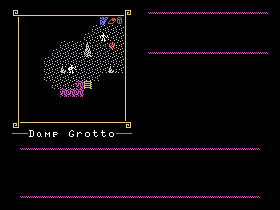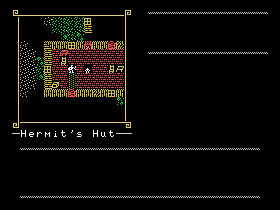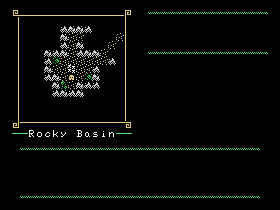## Interlude: A Frustrating Opcode

There's one opcode in particular in TMS9900 assembly language that never fails to annoy me. I've had multiple issues with it in my design efforts, eventually leading to me to use other approaches in most cases.

The opcode? COC, or Compare Ones Cooresponding. (And it's sibling, Compare Zeros Cooresponding for similar reasons.)

The first problem with this opcode is that it has no byte equivalent form. Many times my code has returned a "syntax error" on compilation, because I errantly threw in a COCB... which doesn't exist.

The second problem is that it only allows register-to-register or memory-to-register comparisons. Another error code I kept seeing was "invalid register"... which happens because I tried to do a memory-to-memory compare.

Okay, so everyone makes mistakes. But in this instance, it seems like this particular opcode is really useless in many situations where it could actually be useful.

Consider the normal compare (C) instruction. It has a byte-vector (CB) and it also lets you do all four potential variants of source/destination. (R->R, R->M, M->R, and M->M) It's only natural to assume that COC has this functionality, and yet it doesn't.

Then also consider the SOC and SZC opcodes. (Set Ones and Zeroes Cooresponding) These have byte-vectors. These work on all four variants. So the message here is, be invasive all you want, but you can only passively check it in one manner?

The other issue is when I need to check bit values, the fact I need to use a register means I don't really save much time or memory using COC.

Consider this example. I have a value in the symbolic address @ARRAY+4 that I need to check if the high-bit (>80) is on or off. Using COC, I may do it like this:

```       LI   R1,>8000
MOV  @ARRAY+4,R0
COC  R1,R0
JEQ  ELSEWR
```
Still, it seems wasteful to set up a register (R1) just to get that bit. So you could also do it like this to save an instruction, at the cost of a bit of speed on the COC comparison:
```FILTER DATA >8000

MOV  @ARRAY+4,R0
COC  @FILTER,R0
JEQ  ELSEWR
```
So, there's one way to use COC. But, if you MUST use a register to get the value you want to check, why not do it like this?
```       MOV  @ARRAY+4,R0
ANDI R0,>8000
JNE  ELSEWR
```
Using immediate values is nearly always faster than memory words, because the CPU only has to do a single access back to memory to retrieve the value. For symbolic memory addresses, it has to fetch the address, THEN fetch the value at the address.

Also, I'm taking advantage of the fact that the CPU is doing a comparison every time it performs an operation anyway. If, after AND'ing the value the equality bit in the status register is one, that means the bit wasn't set. Otherwise, it was (thus not equal to zero) and we can do our jump.

About the only time I can see using COC is if you already have a value in a register you need to check, so the move isn't necessary. It is also useful in that it preserves the word being checked, unlike the AND operation above, if you need to check it multiple times.

Still, I've found plenty of better work-arounds. I suppose Texas Instruments must have run out of opcode space, or decided it wasn't necessary. I haven't checked if the 9995 has expanded this particular area of the opcodes or not.

## Conclusion

I'm now moving into display creation for statistics. One of those tasks you think is easy or low-memory, until you start doing it. I'm also going to begin implementing the inventory system, which will be complicated to handle, to say the least. And I'll need my item list before I can really test in earnest...